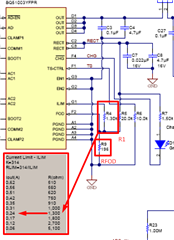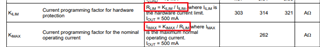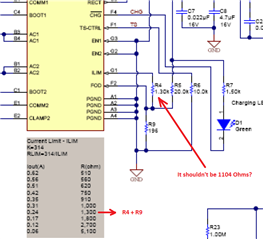# TIDA-00688: Choosing & Setting current limit for bq51003

Part Number: TIDA-00688
Other Parts Discussed in Thread: BQ25100, BQ51003,

Hi everyone, I design a wearable device using the bq51003 wireless receiver and the bq25100 battery charger and I am advising the reference design TIDA-00688. I need your advice regarding the max output current I have to choose for the bq51003  and how to set it.

My wearable has a low current consumption (<5mA in full power mode) and I will use a battery with a capacity 80mAh. The manufacturer suggests using a standard charging method of 0.2C, that is 0.2 x 80mA = 16mA charging current and I have calculated the ISET and PRETERM resistors of bq25100 to meet this requirement.

Given that the bq25100 will draw just around 16-18mA, is means that the bq51003 must provide at least 20mA? I have to take into account and the load as well? For example if the load is 5mA then the total output current of the bq51003 must be at least 35mA?

Now in order to set the limit current

According to the bq51003 datasheet section 9.2.12.5 the default value for RFOD = 196 Ω (the same as the reference design) and the RLIM is calculated as:

RILIM = R1 + RFOD or RILIM = KIMAX / IMAX (see datasheet section 8.3.4)

This is a screenshot from the Reference Design TIDA-00688 section 5.1. The table shows the various resistor values for the corresponding output current IMAX right? As shown from the reference design the R1 = 1.3k corresponding to a maximum output current of 240mA. Using the formulas above I cannot verify the results in the table..

For example:

for IMAX = 0.24A , KIMAX = 262, RFOD = 196Ω

RILIM = KIMAX / IMAX = 262/0.24 = 1092 Ω

R1 = RILIM - RFOD = 1092 - 196 = 896 Ω (for IMAX = 0.24A)

My calculations do not match with the table.. please advice..• Hi Nikos,

The Iout in this case is not intended to be the IMAX. The calculation is for the input limit desired where Iout is not the maximum load . For Iout = 0.240 A, they use Rlim = 314 / 0.240 which comes out to about 1300 Ohms.

Given that the bq25100 will draw just around 16-18mA, is means that the bq51003 must provide at least 20mA? I have to take into account and the load as well? For example if the load is 5mA then the total output current of the bq51003 must be at least 35mA?

For the BQ25100, the current provided will be the total output current that is provided to your load and battery. You will need to compensate for the load power, so yes your estimation of 35 mA should suit your needs.

Best Regards,

Anthony Pham

• Hi and thank you for your responce..

I am still confused.. Could you elaborate a bit more?

In the datasheet there are two equations for RLIM:The first equation is used to allow the wireless IC to source the current limit to the hardware while the second equation is used to allow the wireless IC to source the max current to the hardware right?

Where ILIMIT = 1.2 x IMAX and KILIM = 1.2 x KIMAX

So, it's up to the designer to decide if the wireless IC will supply the max current or the limit current right?

The Iout in this case is not intended to be the IMAX. The calculation is for the input limit desired where Iout is not the maximum load . For Iout = 0.240 A, they use Rlim = 314 / 0.240 which comes out to about 1300 Ohms.

So, basically the Iout is the limit current? The datasheet says that Rlim is the total resistance from the ILIM pin to GND. In the reference design, if Rlim = 1300ohms and R9 = 196 Ohms, then the R4 shouldn't be 1104 Ohms?• Hi Nikos,

Yes it looks like the ILIM that is calculated is based on the IOUT in that table.

The first equation is used to allow the wireless IC to source the current limit to the hardware while the second equation is used to allow the wireless IC to source the max current to the hardware right?

Where ILIMIT = 1.2 x IMAX and KILIM = 1.2 x KIMAX

Yes, this is the relation between ILIM and IMAX. You cannot have design two separate limits without already setting the other.

Looking at the Wireless Receiver portion in the Key System Specifications, the device designed around a 150 mA max load current (1.2 x 150 mA = 180 mA). With the total resistance to ground being 1.3k + 196 = 1.496k, this puts the input current limit at 200mA. This was most likely a design decision to give some headroom.